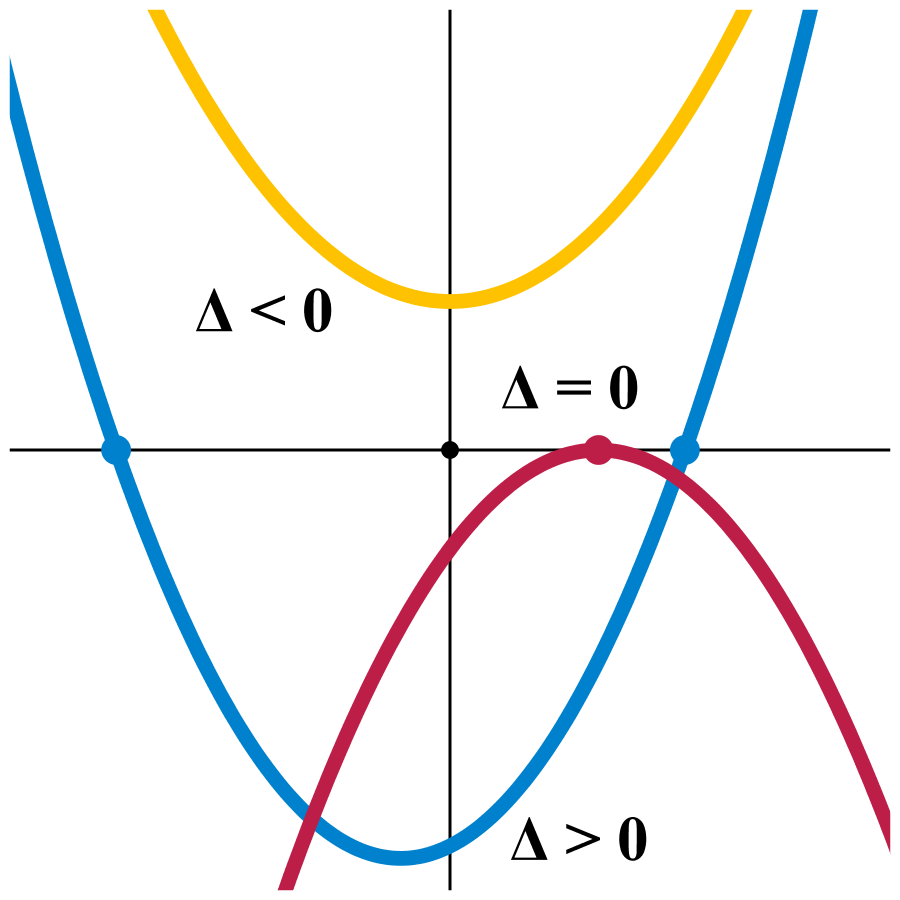# This is the only title I could think ofHow many ordered integer pairs $(a,b)$ satisfy the equation $a^{2} + 3ab - 2b^{2} = 124.$

×